Question

C programming

Write a C program to take N numbers of integer in an array. Then take a number M from user and search it in the array. If number M is found in the array, then print “Number ta paisi!!”, and if not found then print “Pailam na vi number ta!!”.

Sample Input:                                         Sample Output:

Enter value of N: 5

Enter numbers in array: 7 4 5 2 9           Number ta paisi!!

Enter value of M:

#include

int main()

{

// decalre variables

int n,m,arr;

// input value n

printf("Enter value of N: ");

scanf("%d",&n);

// iterate loop and take the value of arr

printf("Enter numbers in array: ");

for(int i=0;i<n;i++)

{

scanf("%d",&arr[i]);

}

// input m

printf("Enter value of M: ");

scanf("%d",&m);

// iterate and check the value present in array

for(int j=0;j<n;j++)

{

if(arr[j]==m)

{

printf("Number ta paisi!!");

return 0;

}

}

// if not then

printf("Pailam na vi number ta!!");

return 0;

}

> Thank you so much

Umar Ali Ashraf Wed, Jan 12, 2022 1:12 AM

Earn Coins

Coins can be redeemed for fabulous gifts.

Similar Homework Help Questions
• use c++ language, keep it simple i am using code block Exercise#2: Arrays with Random Numbers...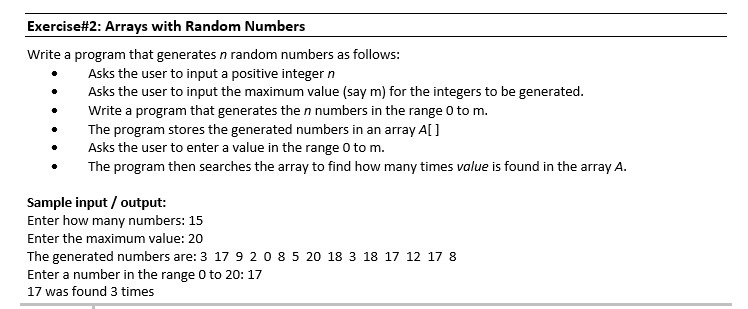use c++ language, keep it simple i am using code block Exercise#2: Arrays with Random Numbers Write a program that generates n random numbers as follows: Asks the user to input a positive integer n Asks the user to input the maximum value (say m) for the integers to be generated. Write a program that generates the n numbers in the range 0 to m. The program stores the generated numbers in an array A[ Asks the user to enter...

• In C++ Please work on the following short programming assignments and upload your solutions on a...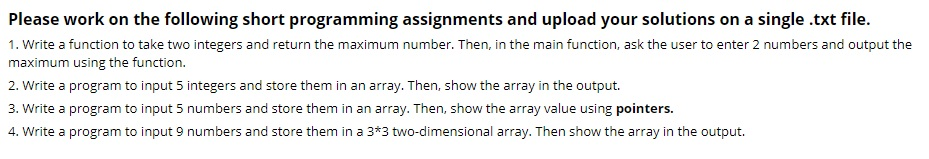In C++ Please work on the following short programming assignments and upload your solutions on a single .txt file. 1. Write a function to take two integers and return the maximum number. Then, in the main function, ask the user to enter 2 numbers and output the maximum using the function. 2. Write a program to input 5 integers and store them in an array. Then, show the array in the output. 3. Write a program to input 5 numbers...

• please solve it before 11:15 today Subject :C++ programming - Lab_9_Sec 51.pdf 1411113: Programming for Engineers...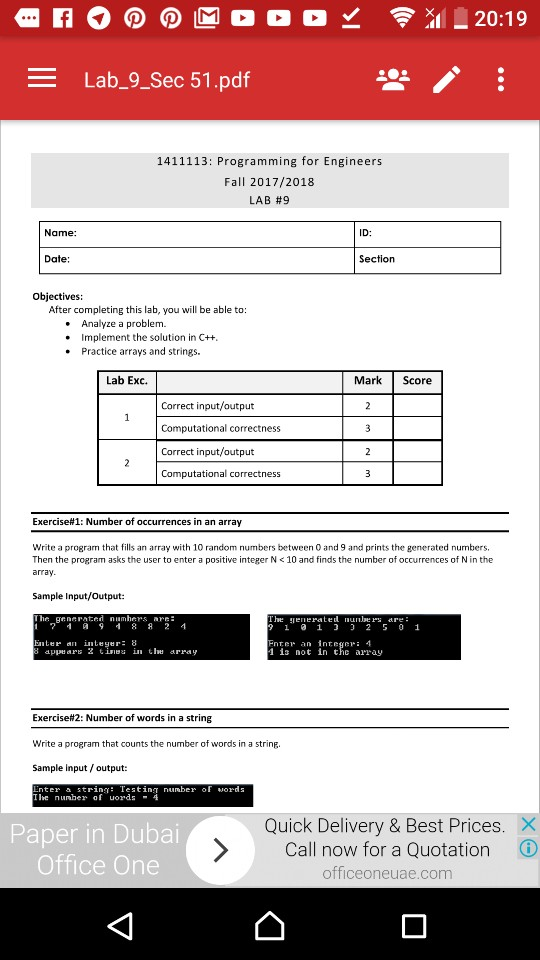please solve it before 11:15 today Subject :C++ programming - Lab_9_Sec 51.pdf 1411113: Programming for Engineers Fall 2017/2018 LAB #9 Name: ID: Date Section Objectives: After completing this lab, you will be able to .Analyze a problem. .Implement the solution in C++ . Practice arrays and strings. Lab Exc. Mark Score Correct input/output Computational correctness Correct input/output Computational correctness Exercise#1: Number of occurrences in an array Write a program that fills an array with 10 random numbers between 0 and...

• c++. please show screenshots of output This project will continue to familiarize the student with using...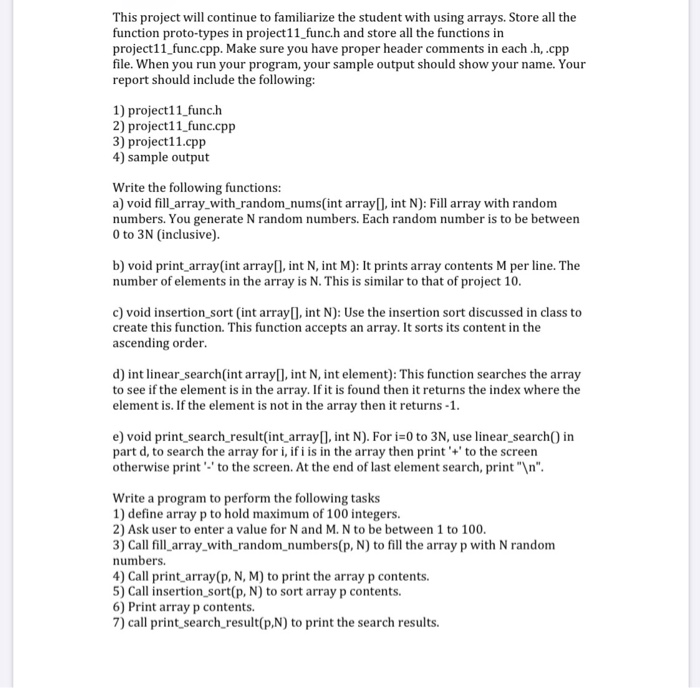c++. please show screenshots of output This project will continue to familiarize the student with using arrays. Store all the function proto-types in project11_funch and store all the functions in project11_func.cpp. Make sure you have proper header comments in each.h,.cpp file. When you run your program, your sample output should show your name. Your report should include the following: 1) project11_func.h 2) project11_func.cpp 3) project11.cpp 4) sample output Write the following functions: a) void fill_array_with_random_nums(int array(), int N): Fill array...

• Use C programming Make sure everything works well only upload Write a program that takes an...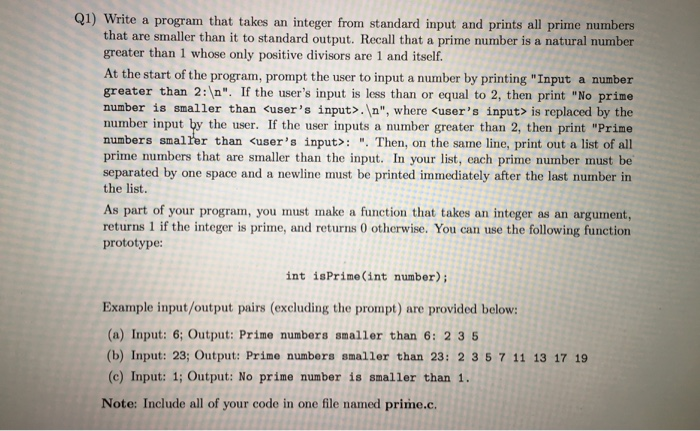Use C programming Make sure everything works well only upload Write a program that takes an integer from standard input and prints all prime numbers that are smaller than it to standard output. Recall that a prime number is a natural number greater than 1 whose only positive divisors are 1 and itself. At the start of the program, prompt the user to input a number by printing "Input a number greater than 2:\n". If the user's input is less...

• LANGUAGE = C i. Write a program that takes int numbers from user until user gives...

LANGUAGE = C i. Write a program that takes int numbers from user until user gives a sentinel value (loop terminating condition). Sort the numbers in ascending order using Insertion sort method. Sorting part should be done in different function named insertionSort(). Your code should count the number of swaps required to sort the numbers. Print the sorted list and total number of swaps that was required to sort those numbers. (4 marks) ii. In this part take another number...

• use C++ programming language and follow the instruction carefully. thanks! Inputs: . [integer] values (10 times)...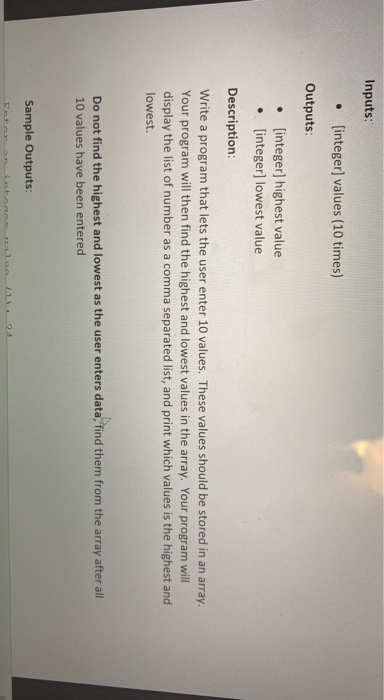use C++ programming language and follow the instruction carefully. thanks! Inputs: . [integer] values (10 times) Outputs: • [integer) highest value . [integer] lowest value Description: Write a program that lets the user enter 10 values. These values should be stored in an array. Your program will then find the highest and lowest values in the array. Your program will display the list of number as a comma separated list, and print which values is the highest and lowest Do...

Write a function that takes in three parameters:an array of integersthe logical size of the arrayan element we're interested in finding in the array (we callthis the search key)The function should go through the array one element at a time,checking each element to see if it's the search key. The functionshould return the indexwhere the search key islocated.Keep in mind that the search key may not be found. Devise anelegant way to return this information.Write a main program that tests...

• Playing With Arrays The following problems are to give you practice with programming with arrays. Write...

Playing With Arrays The following problems are to give you practice with programming with arrays. Write a program to: 1.Ask the user for numbers and place them in an array sequencially. 2.Next, print out the values in the array in the order they are stored. 3.Search the array for the postion containing the smallest value. 4.Print out the position where the smallest value was found. 5.Swap the smallest value with the value in the first postition of the array. 6.Print...

• C- PROGRAMMING PROJECT #4 Design and Write a C program to calculate an average of an...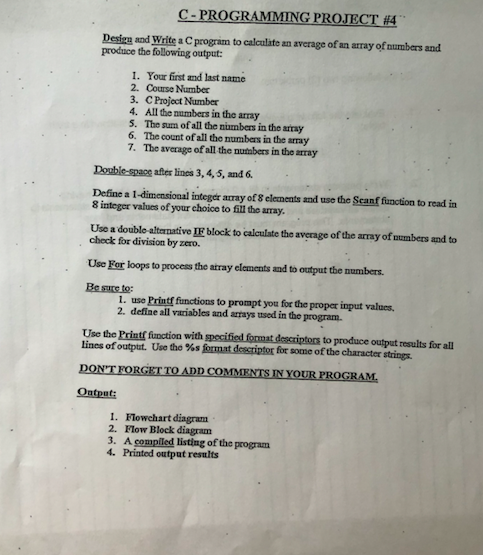C- PROGRAMMING PROJECT #4 Design and Write a C program to calculate an average of an array of numbers and produce the following output: 1. Your first and last name 2. Course Number 3. C Project Number 4. All the numbers in the array 5. The sum of all the numbers in the array 6. The count of all the numbers in the array 7. The average of all the numbers in the array Double-space after lines 3, 4, 5,...